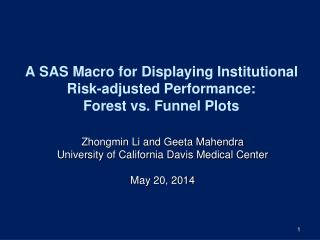DownloadDownload PresentationA SAS Macro for Displaying Institutional Risk-adjusted Performance: Forest vs. Funnel Plots

# A SAS Macro for Displaying Institutional Risk-adjusted Performance: Forest vs. Funnel Plots

Télécharger la présentation## A SAS Macro for Displaying Institutional Risk-adjusted Performance: Forest vs. Funnel Plots

- - - - - - - - - - - - - - - - - - - - - - - - - - - E N D - - - - - - - - - - - - - - - - - - - - - - - - - - -
##### Presentation Transcript

1. A SAS Macro for Displaying Institutional Risk-adjusted Performance: Forest vs. Funnel Plots Zhongmin Li and Geeta Mahendra University of California Davis Medical Center May 20, 2014

2. Overview • Introduction • Data preparation • SAS Macro for Forest vs. Funnel plots • Discussion

3. Introduction • Both forest plot and funnel plot were initially developed for presenting results of meta-analysis • Forest plot: an intuitive, convenient and easily understood display of mean values and their confidence intervals for comparisons • Funnel plots: no spurious ranking; the eye is naturally drawn to important points that lie outside the funnel

4. Data Preparation • 20 observations; one per institution • Variables: • Institutional ID, • total number of cases admitted, • number of adverse events, • observed rate of adverse events, • Expeted rate of adverse event, • Risk adjusted event rate and • the lower and upper limits of 95% confidence interval of the risk adjusted event rate.

5. Sample Data

6. Forest Plot (1) %MACRO ForestPlot (Datain, PopStateRate); … DATA Anno; LENGTH function style color \$8; RETAIN xsys ysys '2' when 'a'; SET &Datain; **Drawing horizontal line for CI of risk-adjusted rate; FUNCTION='move'; xsys='2'; ysys='2'; yc=hospname; x=LL_RAMR; color='black'; output; FUNCTION='draw'; x=UL_RAMR; color='black'; size=1; output;

7. Forest Plot (2) **Drawing tick lines at both ends of the lower and the upper limits Function='move';xsys='2'; ysys='2';yc=hospname; x=ll_RAMR; color='black'; output; Function='draw';x=ll_RAMR; ysys='9'; y=+1; size=1; output; Function='draw';x=ll_RAMR; y=-2; size=1;output; Function='move';xsys='2'; ysys='2'; yc=hospname; x=Ul_RAMR; color='black'; output; Function='draw';x=Ul_RAMR; ysys='9'; y=+1; size=1; output; Function='draw';x=Ul_RAMR; y=-2; size=1; output; RUN;

8. Forest Plot (3) **Create Y axis values in ascending order of the risk adjusted event rate for the graph. PROC SQL is used to create a macro variable HOSP which is then used to order the Y axis labels; PROC SQL noprint; select quote(hospname) into :hosp separated by ' ' from &datain; Quit; Axis1 label=none minor=none offset=(2,2) order=(&hosp); Axis2 order=(0 to 18 by 2) label=('Risk Adjusted Event Rate') minor=none; Symbol1 interpol=none color=black value=dot height=1.5;

9. Forest Plot (4) **Use GPLOT to create the forest plot by plotting hospital name on the Y axis and risk adjusted event rate on the X axis. A vertical reference line for the state population rate is added using the HREF option. PROC GPLOT DATA=&datain; Plot Hospname*adjrate / annotate=anno nolegend Vaxis=axis1 Haxis=axis2 Href = &PopStateRate LHref = 2; Run;   %MEND ForestPlot;

10. Forest Plot: Results

11. Funnel Plot (1) %MACRO FunnelPlot(Datain, MeanProcRate, Sampsize); … **Use SAS IML to generate 95% and 99.8% confidence bands around the average of mean risk adjusted event rate. PROC iml; USE Rpt; READ all var {hospname Voln nobs adjrate se}; CLOSE rpt;

12. Funnel Plot (2) **Create IML module FunnelStat for matrixes. START FunnelStat(x); mean = x[:,]; countn = j(1, ncol(x)); do i = 1 to ncol(x); countn[i] = sum(x[,i]^=.); end; FunnelStat = (x-mean)[##,] / (countn-1); RETURN ( FunnelStat ); FINISH;

13. Funnel Plot (3) **Calculate overall mean, variance and the lower and upper limits for 95% and 99.8% confidence bands for mean risk adjusted event rate; y = adjrate[:]; s = sqrt(FunnelStat(adjrate)); n = T( do(1, &sampsize, 1) ); p = {0.0010.0250.9750.999}; /** lower/upper limits **/ z = quantile("normal", p); limits = y + s / sqrt(n) * z;

14. Funnel Plot (4) **Create a dataset which has all the variables needed to create the funnel plot by combining confidence limits with the rest of the data. d = n || limits; VARNAMES = {"N" "L998" "L95" "U95" "U998" }; CREATE Bounds from d[c=varNames]; APPEND from d; CLOSE Bounds; QUIT; **If statement is used to label only the outliers. DATA plotdata; SET Temp Bounds; outlier=Hospname; if hospname not in ('Unit#8','Unit#15') then outlier=' ';

15. Funnel Plot (5) **Using SGPLOT procedure. PROC SGPLOT DATA=plotdata ; SCATTER x=voln y=adjrate /datalabel=Outlier ; REFLINE &MeanProcRate / axis=y; BAND x=N lower=l95 upper=U95 / nofill name="CI95" LegendLabel="95% limits" lineattrs=(color=gray); … XAXIS label="Procedure Volume Category" fitpolicy=rotate grid values=(1 to 10 by 1) integer ; YAXIS label="Mean Risk-adjusted Event Rate"; KEYLEGEND "CI998" "CI95" / location=inside position=TopRight across=1; … %MEND FunnelPlot;

16. Funnel Plot: Results

17. Discussion • Both Forest and Funnel plots can be used to rate institutions based on their performance. • Forest plots are used more for ranking purposes; Funnel plots are a simple way to compare group means to overall average without ranking • Funnel plots use the point estimates which could be caused by a chance. • Primary audiences for funnel plots are more likely to be researchers and analysts than senior decision makers.